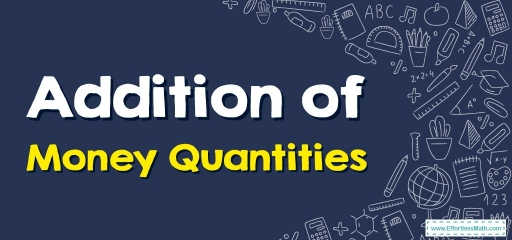Do you know how to add money amounts? This is very simple and we will teach you it in a few simple steps.## Related Topics

Before sending a big bag of cash, you have to be aware of just how much there is at your disposal!

And as it’s spent, you’ll need to be aware of what is getting used. To do that, you must understand how money is identified and be able to do operations like the addition and subtraction of dollars and cents.

Money amounts can be signified in a few varying ways.

Cents can be shown via a $$¢$$ sign and the dollars can be shown via a dollar sign $$()$$.

The addition of money that’s conveyed like this merely involves the addition of the quantities and putting the appropriate sign on your answer.

## How are amounts of money added?

Use these steps to add numbers, together with regrouping! At times, it will help to utilize grid paper to aid you in keeping the digits as well as the decimal points all lined up.

Step one: Line up these numbers: the ones, the tens, the hundreds, and the thousands. You merely need to put a  in front of the $$1$$st number. That tells us the already the other number is likewise in dollars

Step two: Begin doing addition in the column on the right. Move on to the left, plus carry the numbers and regroup as required.

Step three: Write down the answer precisely underneath the column of the corresponding numeral and place a  or a $$¢$$ sign in front of the answer.

### Addition of Money Quantities – Example 1:

$$\begin{array}{r} &334\\ +\!\!\!\!\!&455\\ \hline \end{array}$$

Solution:

First line up the numbers: $$\begin{array}{r} &334\\ +\!\!\!\!\!&455\\ \hline \end{array}→$$ Add the numerals in the ones’ spot column $$(4 + 5 = 9)$$ and put the resulting answer under the line. $$\begin{array}{r} &334\\ +\!\!\!\!\!\!&455 \\ \hline \end{array} \\ \ \ \ \ \ \ \ \ \ \ \ \ 9$$ , Continue with tens’ place. $$3 \ + \ 5=8$$, $$\begin{array}{r} &334\\ +\!\!\!\!\!\!&455 \\ \hline \end{array} \\ \ \ \ \ \ \ \ \ \ \ 8$$. Add the numerals in the hundreds’ spot column then out this answer under the line, $$3 \ + \ 4=7$$, $$\begin{array}{r} &334\\ +\!\!\!\!\!\!&455 \\ \hline \end{array} \\ \ \ \ \ \ \ \ \ 7$$. $$\begin{array}{r} &334\\ +\!\!\!\!\!\!&455 \\ \hline \end{array} \\ \ \ \ \ \ \ 789$$

### Addition of Money Quantities – Example 2:

$$\begin{array}{r} &562\\ +\!\!\!\!\!&233\\ \hline \end{array}$$

Solution:

First line up the numbers: $$\begin{array}{r} &562\\ +\!\!\!\!\!&233\\ \hline \end{array}→$$ Add the numerals in the ones’ spot column $$(2 + 3 = 5)$$ and put the resulting answer under the line. $$\begin{array}{r} &562\\ +\!\!\!\!\!\!&233 \\ \hline \end{array} \\ \ \ \ \ \ \ \ \ \ \ \ \ 5$$ , Continue with tens’ place. $$6 \ + \ 3=9$$, $$\begin{array}{r} &562\\ +\!\!\!\!\!\!&233 \\ \hline \end{array} \\ \ \ \ \ \ \ \ \ \ \ 9$$. Add the numerals in the hundreds’ spot column then out this answer under the line, $$5 \ + \ 2=7$$, $$\begin{array}{r} &562\\ +\!\!\!\!\!\!&233 \\ \hline \end{array} \\ \ \ \ \ \ \ \ \ 7$$. $$\begin{array}{r} &562\\ +\!\!\!\!\!\!&233 \\ \hline \end{array} \\ \ \ \ \ \ \ 795$$

## Exercises for the Addition of Money Quantities

1. $$\color{blue}{784+525}$$
2. $$\color{blue}{963+159}$$
3. $$\color{blue}{847+452}$$
4. $$\color{blue}{673+354}$$
1. $$\color{blue}{1,309}$$
2. $$\color{blue}{1,122}$$
3. $$\color{blue}{1,299}$$
4. $$\color{blue}{1,027}$$

### What people say about "Addition of Money Quantities - Effortless Math: We Help Students Learn to LOVE Mathematics"?

No one replied yet.

X
30% OFF

Limited time only!

Save Over 30%

SAVE $5 It was$16.99 now it is \$11.99